# AP Board 9th Class Maths Solutions Chapter 8 Quadrilaterals Ex 8.3

AP State Syllabus AP Board 9th Class Maths Solutions Chapter 8 Quadrilaterals Ex 8.3 Textbook Questions and Answers.

## AP State Syllabus 9th Class Maths Solutions 8th Lesson Quadrilaterals Exercise 8.3

Question 1.
The opposite angles of a parallelogram are (3x – 2)° and (x + 48)°. Find the measure of each angle of the parallelogram.
Solution:
Given that the opposite angles of a parallelogram are (3x – 2)° and (x + 48)°
Thus 3x – 2 = x + 48
(∵ opp. angles of a //gm are equal)
3x – x = 48 + 2
2x = 50
x = $$\frac{50}{2}$$ = 25°
∴ The given angles are (3 x 25 – 2)° and (25 + 48) °
= (75 – 2)° and 73° = 73° and 73°
We know the consecutive angles are supplementary.
∴ The other two angles are (180°-73°) and (180°-73°)
= 107° and 107°
∴ The four angles are 73°, 107°, 73° and 107°.Question 2.
Find the measure of all the angles of a parallelogram, if one angle is 24° less than the twice of the smallest angle.
Solution:
Let the smallest angle = x
Then its consecutive angle = 180 – x°
By problem (180 – x)° = (2x- 24)°
(∵ opp. angles are equal)
180 + 24 = 2x + x
3x = 204
x = $$\frac{204}{3}$$ = 68°
∴ The angles are
68°; (2 x 68 – 24)°; 68°; (2 x 68 – 24)°
= 68°, 112°, 68°, 112°

Question 3.
In the given figure ABCD is a paral-lelogram and E is the mid point of the side BC. If DE and AB are produced to meet at F, show that AF = 2AB.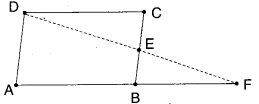Solution: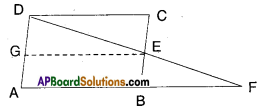Given that □ABCD is a parallelogram.
E is the midpoint of BC.
Let G be the midpoint of AD.
Join G, E.
Now in ΔAFD, GE is the line joining the midpoints G, E of two sides AD and FD.
∴GE // AF and GE = $$\frac{1}{2}$$AF
But GE = AB [ ∵ ABEG is a parallelo¬gram and AB, GE forms a pair of opp. sides]
$$\frac{1}{2}$$ = AB ⇒ AF = 2AB
Hence Proved.Question 4.
In the given figure ABCD is a paral¬lelogram. P, Q are the midpoints of sides AB and DC respectively. Show that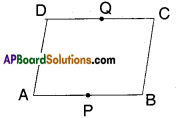Solution: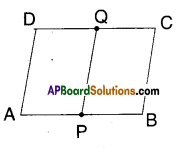□ABCD is a parallelogram.
P, Q are the mid points of AB and CD.
Join Q, P.
Now AB = CD (Opp. sides of a //gm)
$$\frac{1}{2}$$AB = $$\frac{1}{2}$$CD
PB = QC
Also PB // QC.
Now in □PBCQ;
PB = QC; PB//QC
Hence □PBCQ is a parallelogram.

Question 5.
ABC is an isosceles triangle in which AB = AC. AD bisects exterior angle QAC and CD//BA as shown in the figure. Show that i) ∠DAC = ∠BCA
ii) ABCD is a parallelogram.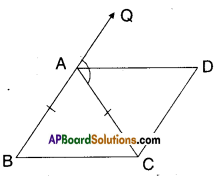Solution:
Given that AABC is isosceles; AB = AC

i) In ΔABC, AB = AC ⇒ ∠B = ∠ACB
(angles opp. to equal sides)
Also ∠QAC = ∠B + ∠ACB
∠QAC = ∠BCA + ∠BCA
(∵∠BCA = ∠B)
⇒ $$\frac{1}{2}$$∠QAC = $$\frac{1}{2}$$ [2 ∠BCA]
⇒ ∠DAC = ∠BCA [ ∵ AD is bisector of ∠QAC]ii) From (i) ∠DAC = ∠BCA
But these forms a pair of alt. int. angles for the pair of lines AD and BC; AC as a transversal.
In □ABCD ; AB // DC; BC // AD
□ABCD is a parallelogram.

Question 6.
ABCD is a parallelogram AP and CQ are perpendiculars drawn from vertices A and C on diagonal BD (see figure). Show that 1) ΔAPB ≅ ΔCQD ii) AP = CQ.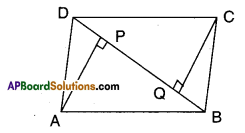Solution:
Given that □ABCD is a parallelogram.
BD is a diagonal.
AP ⊥ BD and CQ ⊥ BD
i) In ΔAPB and ΔCQD
AB = CD ( ∵ Opp. sides of //gm ABCD)
∠APB = ∠CQD (each 90°)
∠PBA = ∠QDC (alt. int. angles for the lines AB and DC)
∴ ΔAPB ≅ ΔCQD (AAS congruence)

ii) From (1) ΔAPB ≅ ΔCQD
⇒ AP = CQ (CPCT)Question 7.
In Δs ABC and Δs DEF, AB = DC and AB//DE; BC = EF and BC//EF. Vertices A, B and C are joined to vertices D, E and F respectively (see figure). Show that
i) ABED is a parallelogram
ii) BCFE is a parallelogram
iii) AC = DF
iv) ΔABC = ΔDEF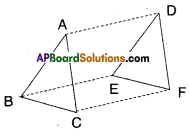Solution: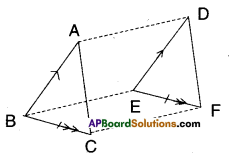Given that in ΔABC and ΔDEF
AB = DE and AB // DE
BC = EF and BC//EF.
i) In □ABED AB//ED and AB = ED
Hence □ABED is a parallelogram.

ii) In □BCFE; BC = EF and BC//EF
Hence □BCFE is a parallelogram.

iii) ACFD is a parallelogram (In a paral-lelogram opposite sides are equal).
So, AC = DF.

iv) Consider ΔABC = ΔDEF
AB = DE (given);
AC = DF (proved)
BC = EF (given)
∴ ΔABC ≅ ΔDEF (SSS congruency rule).

Question 8.
ABCD is a parallelogram. AC and BD are the diagonals intersect at ‘O’. P and Q are the points of trisection of the diagonal BD. Prove that CQ//AP and also AC bisects PQ.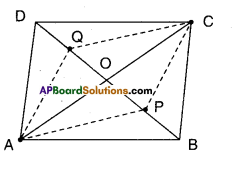Solution:
Given □ABCD is a parallelogram;
BD is a diagonal.
P, Q are the points of trisection of BD.
In ΔAPB and ΔCQD
AB = CD (•.• Opp. sides of //gm ABCD)
BP = DQ (given)
∠ABP = ∠CDQ (alt. int. angles for the lines AB//DC, BD as a transversal)
ΔAPB = ΔCQD (SAS congruence)
Similarly in ΔAQD and ΔCPB
AD = BC (opp. sides of //gm ABCD)
DQ = BP (given)
∠ADQ = ∠CBP (all int. angles for the lines AD//BC, BD as a transversal)
ΔAQD ≅ ΔCPB
Now in □APCQ
AP = CQ (CPCT of AAPB, ACQD)
AQ = CP (CPCT of AAQD and ACPB)
∴ □APCQ is a parallelogram.
∴ CQ//AP (opp. sides of//gm APCQ)
Also AC bisects PQ. [ ∵ diagonals of //gm APCQ]Question 9.
ABCD is a square. E, F, G and H are the mid points of AB, BC, CD and DA respectively. Such that AE = BF = CG = DH. Prove that EFGH is a square.
Solution: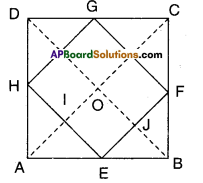Given that ABCD is a square.
E, F, G, H are the mid points of AB, BC, CD and DA.
Also AE = BF = CG = DH
In ΔABC; E, F are the mid points of sides AB and BC.
∴ EF//AC and EF = $$\frac{1}{2}$$ AC
Similarly GH//AC and GH = AC
GF//BD and GF = $$\frac{1}{2}$$ BD
HE//BD and HE = $$\frac{1}{2}$$ BD

But AC = BD (∵ diagonals of a square)
∴ EF = FG = GH = HE
Hence EFGH is a rhombus.
Also AC ⊥ BD
(∵ diagonals of a rhombus)
∴ In //gm OIEJ [ ∵ 0I // EJ; IE // OJ]
We have ∠IOJ = ∠E
[ ∵ Opp. angles of a //gm]
∴ ∠E – 90°
Hence in quad. EFGH; all sides are equal and one angle is 90°.
∴ EFGH is a square.